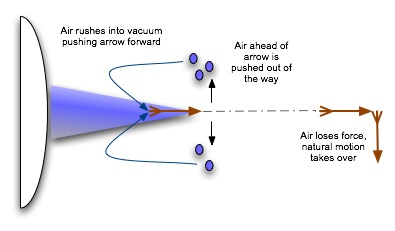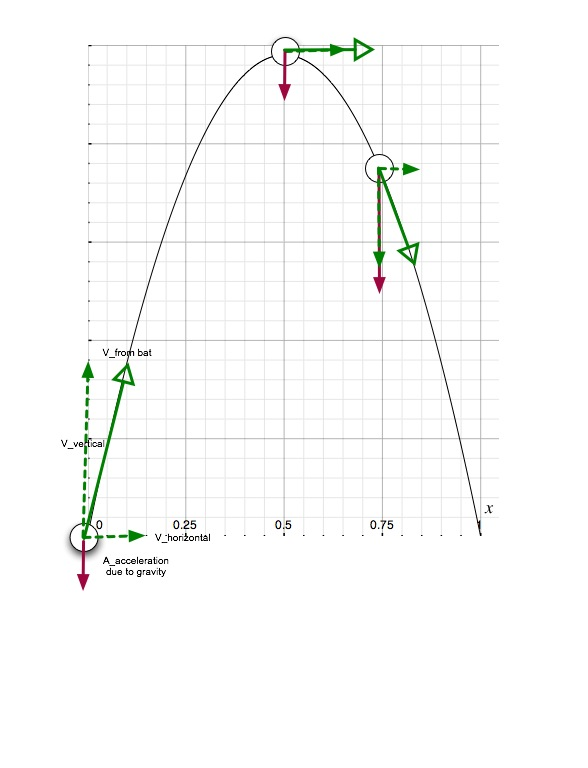# Natural Science - Year I

#### Unit 32: Newton and Universal LawScience Weblecture for Unit 32

 This Unit's Homework Page History Lecture Science Lecture Lab Parents' Notes

# Science Lecture for Unit 32: The Law of Gravity

### For Class

• Topic area: Physics: Forces, gravity
• Terms and concepts to know: constant acceleration, changing acceleration, projectile motion
• See historical period(s): Universal Law

Lecture Outline

We now reach the mid-point of this course: the end of the Scientific Revolution and the beginning of "modern science". We've covered theories of matter and motion from the Greeks to the seventeenth century, and looked at both living and nonliving systems, earth-based and terrestrial systems, and things we describe with and without mathematics. Over this period, we've been constantly moving toward more quantifiable methods of science. We can do a number of things that we couldn't do when we first started looking at the weather.

#### Projectile MotionRemember Aristotle's different types of matter? Earth was attracted to "the center", water was also attracted to the center but as strongly. Both earth and water had gravitas. Air, on the other hand, naturally moved away from the center, and fire moved away even more rapidly. Both air and fire had levitas. If we wanted to move an object like an arrow some direction other than down, we had to push it with a force to give it violent motion, and when the source of the force was withdrawn and the force had spent itself, the object would resume its natural motion (usually down toward the center, again).

We don't actually observe this kind of behavior with projectiles. Anyone who has watched a baseball sail out of the the park on a long homerun when the wind is still can tell you that the ball moves straight out toward center field with about the same speed, but rises and falls on the way. In modern motion analysis, we represent vector quantities like force and velocity with arrows. The size of the arrow indicates magnitude; the arrow points in the direction of the vector.In this diagram, the force of gravity is represented by the maroon arrow. It always points down, with the same magnitude (since gravitational acceleration doesn't change much with small changes in distance from the center of the earth). Velocity is represented by the open-headed green arrow. To make it easier to see the effect downward acceleration has on velocity, we break the velocity vector into its vertical (up/down) and horizontal (forward) components. The horizontal component in this case doesn't change, because there is no force operating on that component of the ball's motion. But the acceleration due to gravity does affect the upward velocity of the ball, causing it to slow down, stop, and then fall back to earth.

The ball hits the hand of the waiting fan with close to the same horizontal speed that it started with. This speed is governed by the law of inertia that says the ball will continue in its forward motion without change. The only "outside" force operating on the horizontal motion of the ball is air resistance, and that doesn't slow the ball down much.

The up and down motion is governed by the law of gravity that says the ball is under constant downward acceleration due to the attractive force between the ball and the earth. The hitter hits the ball up. The acceleration down causes the ball to slow down in the vertical direction, stop (vertical velocity equals zero), and then fall back to earth with increasing vertical velocity, since the velocity now points along the same direction as the acceleration resulting from gravitational forces. Note that we use the term acceleration to mean any change in velocity: it can be an increase or decrease in speed, or a change in direction, or both a change in speed and direction.

In Newton's mechanical universe, if we know the speeds at which the ball was traveling up and out, the acceleration of the ball's horizontal and vertical speeds due to air resistance (often negligible), and the acceleration in the vertical direction due to gravity, we can predict the flight of the ball. It is this power of prediction that gives classical mechanics (sometimes called Newtonian mechanics) its importance in modern science. Armed with a few basic pieces of information, we can build a machine that will toss a ball at a batter for pitching practice, or lob a spacecraft at where Mars will be in six months, and hit our target.

• What happens if a cannonball is shot with a velocity such that the earth's gravity can't pull it back down to the earth's surface?
• What is the center of mass?
• Use the Kepler's Laws Calculator (under Two Limiting Cases) to determine what the period is if you have two masses of 5.0 and 3.0 solar masses each, at a separation of 20.00AU. What happens if you increase the smaller mass to 6.0 solar masses? Is the period now larger or smaller than it was before? Why do you think this happens?

### Prediction and Causality

In science, we are constantly looking for patterns: motions that repeat, events that happen the same way, relationships that hold for different pairs of objects. Kepler found such a pattern when he determined that the periods of planets orbiting the sun were related to their distance from the sun in a particular way. If we use P for period in years and A for distance in astronomical units (the distance between the earth, which takes 1 year to orbit the sun, and the sun), this relationship is P2 = A3

Kepler showed that this worked for each of the visible planets, but he couldn't explain why.

Newton's law of gravity and his laws of motion provides the cause for this pattern. Remember that an object moving in a circle, even if it isn't changing its speed or period, must be under an outside force because it is changing direction. This force (regardless of how it arises -- it could be from gravity, electrical charge, or a string pulling on a ball) is related to the mass of the object m, its distance r from the center of its orbit, and its speed v along its orbit as: Fcentripetal = mv2/r

Newton's law of gravity says that the force of gravity is the product of the two masses M and m, the distance d between the masses, and a constant of proportionality G that is the same in all situations: Fgravity = GMm/r2

We can now put this information together and show that Kepler's law relating the periods of the planets to their distance from the sun arises from the nature of the forces that keep them in orbit.

#### The Derivation of Kepler's Laws: the movie

Here's the derivation. (NO, you do not have to memorize it....until you take Physics). Click HERE to run a Quicktime movie of the derivation. The movie is set up so that you can click when you want it to proceed to the next step. Some pages will require you to click several times while an equation is built.

### Study/Discussion Questions:

• How does the ability to predict the outcome of a situation shape the way we do science?
• Gravity is much weaker than electricity and magnetism, but it is gravity and not electrical or magnetic forces that governs the structure of the universe. What characteristic of gravity makes this possible?